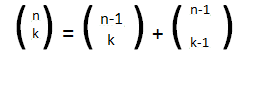# GATE | GATE CS 1997 | Question 59

Consider the following function

```Function F (n, m: integer): integer;
begin
If (n<=0) or (m<=0) then F:=1
else
F:= F(n-1,m) + F(n, m-1);
end;```

Use the recurrence relation to answer the following question.Assume that n, m are positive integers. Write only the answers without any explanation.
a. What is the value of F(n,2)?
b. What is the value of (n,m)?
c. How many recursive calls are made to the function F, including the original call, when evaluating F(n,m).

Explanation:

Quiz of this Question

My Personal Notes arrow_drop_up
Article Tags :

Be the First to upvote.

Please write to us at contribute@geeksforgeeks.org to report any issue with the above content.